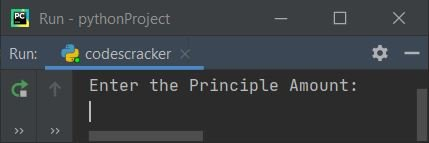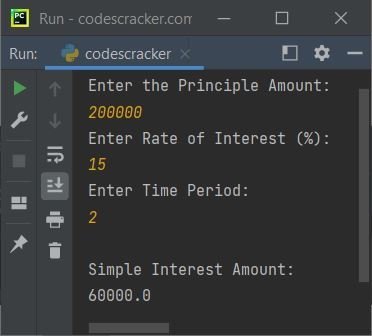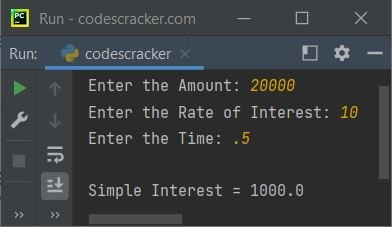# Python Program to Calculate Simple Interest

In this article, you will learn and get code in Python to calculate and print simple interest based on user inputs. Here is a list of programs of simple interest using Python:

• Calculate simple interest without using a function.
• Calculate simple interest using a user-defined function.

But before going through these programs, let's understand the formula used to calculate simple interest.

### Simple Interest Formula

To find simple interest, use the formula given below:

`SI = (P*R*T)/100`

Here SI indicates simple interest, P indicates principle amount (deposited or loan amount), R indicates rate of interest (%), and T indicates time (in years). If the time period of the loan or investment is provided in months, then multiply 100 by 12. That is, we've got to enter the time T in years. Therefore, for 2 months, the year will be 2/12, 1/6, or 0.166666666.

## Calculate Simple Interest in Python

This program calculates and prints simple interest based on the principle, rate of interest, and year provided by the user at runtime. The question is: write a program in Python to calculate simple interest. Here is its answer:

```print("Enter the Principle Amount: ")
p = int(input())
print("Enter Rate of Interest (%): ")
r = float(input())
print("Enter Time Period: ")
t = float(input())
si = (p*r*t)/100
print("\nSimple Interest Amount: ")
print(si)```

Here is its sample run:Now supply inputs, say 200000 as the principle amount, 15 as the rate of interest, and 2 as years. After supplying all inputs, press the ENTER key to find and print the value of simple interest, as shown in the snapshot given below:## Calculate simple interest using the function

This program does the same job as the previous program, but uses a user-defined function named calculateSI(). The function takes three arguments and returns SI. So we've passed all three inputs from the user as arguments, and the return value gets assigned to si. Prints si on output; that is the answer to simple interest.

```def calculateSI(a, b, c):
return (a*b*c)/100

print(end="Enter the Amount: ")
p = int(input())
print(end="Enter the Rate of Interest: ")
r = float(input())
print(end="Enter the Time: ")
t = float(input())
si = calculateSI(p, r, t)
print("\nSimple Interest = " + str(si))```

Here is its sample run with user input: 20000 as amount, 10 as interest rate, and .5 (1/2 years or 6 months) as time:Python Online Test

« Previous Program Next Program »